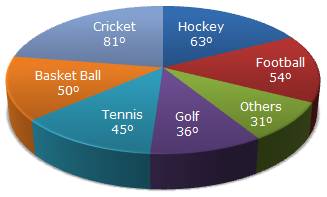# Data Interpretation - Pie Charts

### Exercise :: Pie Charts - Pie Chart 9

The circle-graph given here shows the spendings of a country on various sports during a particular year. Study the graph carefully and answer the questions given below it.1.

What percent of total spending is spent on Tennis?

A.
 12 1 % 2
B.
 22 1 % 2
C. 25%
D. 45%

Explanation:

 Percentage of money spend on Tennis =45 x 100% = 12 1 %. 360 2

2.

How much percent more is spent on Hockey than that on Golf?

 A. 27% B. 35% C. 37.5% D. 75%

Explanation:

Let the total spendings on sports be Rs. x. Then,

 Amount spent on Golf = Rs.36 x x= Rs. x . 360 10

 Amount spent on Hockey = Rs63 x x= Rs. 7x . 360 40

 Difference = Rs.7x - x= Rs 3x . 40 10 40Required percentage = Rs.3x/40x 100% = 75%. x/10

3.

If the total amount spent on sports during the year be Rs. 1,80,00,000 , the amount spent on Basketball exceeds on Tennis by:

 A. Rs. 2,50,000 B. Rs. 3,60,000 C. Rs. 3,75,000 D. Rs. 4,10,000

Explanation:

Amount spent on Basketball exceeds that on Tennis by:

 Rs.(50 - 45) x 1,80,00,000= Rs. 2,50,000. 360

4.

How much percent less is spent on Football than that on Cricket?

A.
 22 2 % 9
B. 27%
C.
 33 1 % 3
D.
 37 1 % 7

Explanation:

Let the total spendings on sports be Rs. x. Then,

 Amount spent on Cricket = Rs.81 x x= Rs. 9x . 360 40

 Amount spent on Football = Rs54 x x= Rs. 3x . 360 20

 Difference = Rs.9x - 3x= Rs 3x . 40 20 40Required percentage = Rs.3x/40x 100% = 33 1 %. 9x/40 3

5.

If the total amount spent on sports during the year was Rs. 2 crores, the amount spent on Cricket and Hockey together was:

 A. Rs. 8,00,000 B. Rs. 80,00,000 C. Rs. 1,20,00,000 D. Rs. 1,60,00,000

Explanation:

Amount spent on Cricket and Hockey together
 = Rs.(81 + 63) x 2crores 360
= Rs. 0.8 crores
= Rs. 80,00,000.